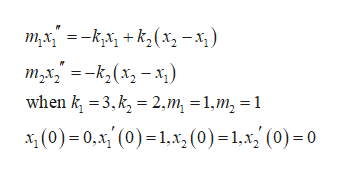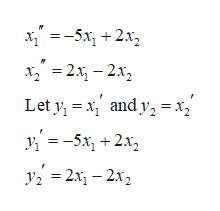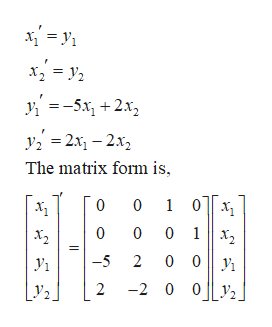# Solve the given system:m1x1''=-k1x1+k2(x2-x1)m2x2''=-k2(x2-x1)when k1=3, k2=2, m1=1, m2=1 and x1(0)=0, x1'(0)=1, x2(0)=1, x2'(0)=0x1(t)=x2(t)=

Question
8 views

Solve the given system:

m1x1''=-k1x1+k2(x2-x1)

m2x2''=-k2(x2-x1)

when k1=3, k2=2, m1=1, m2=1 and

x1(0)=0, x1'(0)=1, x2(0)=1, x2'(0)=0

x1(t)=

x2(t)=

check_circle

Step 1

It is given that, the system and the initial conditions are,help_outlineImage Transcriptionclosem,x=-k,x +k2(x,-x when k 3,k 2,m =1,m2 =1 (0)1,, (0) =1,x, (0) = 0 (0)0 fullscreen
Step 2

Substitute the initial conditions as,help_outlineImage Transcriptionclose--5x, + 2х, x22-2x2 and y x у 3-5х, + 2х, y2 2x-2x2 - 2х, — 2х, fullscreen
Step 3

The first order system o...help_outlineImage Transcriptionclose5x2x y2x-2x2 The matrix form is 0 1 0 0 0 0 1 0 0 -5 2 |y2. 2 2 0 0 fullscreen

### Want to see the full answer?

See Solution

#### Want to see this answer and more?

Solutions are written by subject experts who are available 24/7. Questions are typically answered within 1 hour.*

See Solution
*Response times may vary by subject and question.
Tagged in

### Math